Accept

## Practical application of the Android Neural Network API for the use of Tensorflow Lite models## Introduction

Interest in artificial intelligence is growing every year, and the computing power of devices is growing in proportion to the trend. Huawei has unveiled in London a new family of flagship models, the Mate 20, with a new neural processor (Dual-NPU) with the announced double performance gain. For us at the moment, most mobile phones cannot perform image analysis in Real Time for complex neural network architectures. To solve this problem, there are many methods, quantization of weights, the use of optimized libraries for specific processors or the use of a graphic neural processor. In the case of the first method, quantization of weights has a positive effect on the performance of the application, but after improving the performance, it is worth noting that the quality decreases and in tasks where the quality of work is the priority, this method is not suitable. Not so long ago, in most applications that used neural networks, only CPU resources were used.

One of the first applications to use a graphics processor is the “Prisma” application. In the first releases, the application used cloud computing. Later on, the Prisma development team made custom support for the use of a graphics processor, which removed the server rent from expenses and transferred all the calculations locally to devices. With time, frameworks for optimizing CPU performance began to appear. NCNN, NNPACK and others could be such an example. Recently, the Android Team itself released API 27 which includes the Neural Networks API (NNAPI for short) and it will be discussed in this article.

## What is NNAPI?

As the site with the documentation states: 

The Android Neural Networks API (NNAPI) is an Android C API. It is designed to provide a higher level training system (for example, TensorFlow Lite, Caffe2, or others). The API is available on all devices running Android 8.1 (API level 27) or higher. ”

Simply put, NNAPI will be called by libraries, frameworks and machine learning tools that allow developers to train their models outside the device and deploy them on Android devices. Typically, applications will not use NNAPI directly, but instead will use high-level machine learning systems. These structures, in turn, can use NNAPI to perform hardware-accelerated output operations on supported devices.

Based on the requirements of the application and the hardware capabilities of the device, the operating time of the neural networks of Android can effectively distribute the workload of the calculations through the available processors on the device, including specialized neural network hardware, graphics processors (GPU) and digital signal processors (DSP). For devices that lack a specialized supplier driver, the NNAPI runtime uses optimized code to execute queries on the CPU.

Figure 1 shows a high-level system architecture for NNAPI.

## Description of the basic NNAPI tools.

To work with NNAPI, you first need to build a directed graph that defines the calculations to be performed. This graph of calculations in combination with your input data (weights and bias) forms the model. The constructed model can be used further by passing it input data and receiving the answer at the output. In order to build this graph we have the following tools:

Operands:

Operands are data objects used in determining the graph. These include the inputs and outputs of the model, intermediate nodes that contain data that comes from one operation to another, and constants that are passed to these operations. There are two types of operands that can be added to NNAPI models: scalars and tensors.

To define an operand, you first need to determine its type. For this you need:

``````ANeuralNetworksOperandType tensor3x4Type;
tensor3x4Type.type = ANEURALNETWORKS_TENSOR_FLOAT32; // Select the type of operands
tensor3x4Type.scale = 0.f; // These fields are useful for quantized tensors.
tensor3x4Type.zeroPoint = 0; // These fields are useful for quantized tensors.
tensor3x4Type.dimensionCount = 2;
uint32_t dims  = {3, 4};
tensor3x4Type.dimensions = dims;``````

Using the method ANeuralNetworksModel_addOperand(model, & tensor3x4Type) you can add an operand. The order of adding the operand does not matter, but you must know the indices of the operand used in the operation.

Operations

An operation specifies the computations to be performed. Each operation consists of these elements:

• an operation type (for example, addition, multiplication, convolution),
• a list of indexes of the operands that the operation uses for input and
• a list of indexes of the operands that the operation uses for output.

A full list of operations supported by NNAPI can be obtained by reference.

## Building network architecture.

Since NNAPI does not support network training, we’ll use weights from a pre trained tf lite model. This article focuses on the NNAPI part, so we won’t describe the process here, check out tensorflow documentation and tf lite doc on how to do this. In order for your network to work, you’ll need your network architecture to be exactly as outlined in figure 2 in order for it to match the NNAPI operations we use in the article. Exemplary trained weights that are used in this article can be downloaded from here.

As an example, we will construct a graph of calculations for a trained network on the MNIST dataset, in the format of tensorflow lite, the trained weights will convert the weights into a binary format and loaded into the corresponding operands.

For our examples, a network with two convolutional layers and two fully connected layers with softmax at the output was used.

In the next step, we need to build a computation graph similar to the trained model in the tensorflow lite, adding the appropriate operands and operations by calling ANeuralNetworksModel_addOperation ().

As parameters of this call, your application should provide:

• type of operation,
• number of input values,
• array of indices for input operands,
• number of output values and
• array of indexes for output operands.

``````ANeuralNetworksModel_addOperation (model_, ANEURALNETWORKS_DEPTHWISE_CONV_2D, 8, Input_operands_0, 1, output_operands_0);
ANeuralNetworksModel_addOperation (model_, ANEURALNETWORKS_MAX_POOL_2D, 7, input_operands_1, 1, output_operands_1);
ANeuralNetworksModel_addOperation (model_, ANEURALNETWORKS_CONV_2D, 7, input_operands_2, 1, output_operands_2);
ANeuralNetworksModel_addOperation (model_, ANEURALNETWORKS_MAX_POOL_2D, 7, input_operands_3, 1, output_operands_3);
ANeuralNetworksModel_addOperation (model_, ANEURALNETWORKS_FULLY_CONNECTED, 4, input_operands_4, 1, output_operands_4);
ANeuralNetworksModel_addOperation (model_, ANEURALNETWORKS_FULLY_CONNECTED, 4, input_operands_5, 1, output_operands_5);
ANeuralNetworksModel_addOperation (model_, ANEURALNETWORKS_SOFTMAX, 2, input_operands_6, 1, output_operands_6);
``````

## Loading the weights of the tensorflow lite model into the NNAPI graph.

As discussed in the previous section, we build a graph in NNAPI identical to the graph previously constructed and trained in tensorflow. After our computational model graph is constructed, we need to initialize the operands with our values. In order to get the tensorflow lite binary file format, we will use the Neural Network Transpiler tool. This utility allows you to generate a binary file from a tflite file, and also generates related files in C ++ for use in NNAPI. In my case the generated files had a lot of errors, so I would advise you to use code written by yourself or use it as an aid in writing the architecture yourself.

To convert the weights into a binary format, the following needs to be done in the project directory.

In a project directory create a build directory

`\$ mkdir build\$ cd build`

In build directory call cmake to generate the make file, and call the make program

`\$ cmake ..\$ make`

Create in build directory folder mobnet_path

`\$ mkdir mobnet_path `

Сopy the neural network to the build directory and execute the command:

` \$ ./nnt -m your_networks_name.tflite -j com.nnt.nnexample -p mobnet_path`

At the next stage in the binary file it was necessary to determine how the weights and offsets are recorded. At this stage, I had some problems, because I did not know in what order the weights and displacements in the binary file for each of the layers were recorded. To solve this problem, I did the following. The idea is to find out the first values ​​for each layer and by knowing the size of the layer we can find out its latest index in the binary file. The first value for each layer can be found using the viewer for neural network.

Knowing the first value, we can recognize its index by iterating over the entire binary file. To do this, we run the script using the NumPy library and iterate over each value in the array.

``````import numpy as np
arr = np.fromfile ('weigths_biases.bin', dtype = np.float32)
for index_weigths in range (len (arr)):
if weigths_from_neutron == arr [index_weigths]:
print (index_weigths)``````

After we know the initial indices for each weight and bias, we can load the weights into the corresponding operands. Create an ANeuralNetworksMemory instance by calling the ANeuralNetworksMemory_createFromFd () function, and passing the data file to the file descriptor. You can also specify memory protection flags and an offset where the shared memory area begins in the file.

``````off_t offset, length;
int fd = AAsset_openFileDescriptor (asset, & offset, & length);
ANeuralNetworksMemory_createFromFd (length + offset, protect, fd, 0,
& memoryModel_);``````

Create an instance of AneuralNetworksMemory, from it we can load the weight in the operands, making this method:

``ANeuralNetworksModel_setOperandValueFromMemory (model_, 2,memoryModel_, offset_, tensor_size)``

As the parameters of this call, your application must provide:

• operands index,
• the memory buffer,
• the offset and
• operand length.

Determine which operands the model should consider as its inputs and outputs, calling the ANeuralNetworksModel_identifyInputsAndOutputs () function. And compile the model.

``````ANeuralNetworksModel_identifyInputsAndOutputs (model_, 1, input_indexes, 1, output_indexes);
ANeuralNetworksModel_finish (model_);
ANeuralNetworksCompilation_create (model_, & compilation);
ANeuralNetworksCompilation_setPreference (compilation, ANEURALNETWORKS_PREFER_LOW_POWER);
ANeuralNetworksCompilation_finish (compilation);``````

You can optionally influence how the runtime environment works by using battery power and speed of execution. You can do this by calling ANeuralNetworksCompilation_setPreference ().

## Conclusions

In this way, you can program any tensorflow lite network in your application, do mathematical calculations on tensors, change the weights of the trained network, get information about the calculations on each of the layers, or use multiple neural networks in one graph of calculations and so on. This article reveals the basic features of the NNAPI tool. It is also worth noting the advantage of writing drivers for the distribution of resources used; depending on the type of calculations, that is, you can allocate more computing resources to a higher priority task than to a lower priority one. It is worth remembering that in the absence of specialized drivers on the device, the application will use optimized code to perform requests on the CPU.Andrei Liudkievich

AI Dev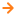Previous
All posts
Next## Interested in collaborating?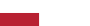Codahead implements a project co-financed from European Funds "Promotion of services provided by Codahead in selected foreign markets." The aim of the project is to develop the company's export activities in foreign markets.

## Get an Estimate

####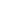Rydlówka 20, 30-363 Kraków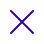What would you like to do?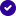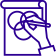Build something new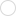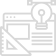Improve existing project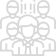Extend my team

What is the scope of your project?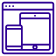Software Development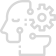AI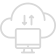IoT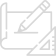Product Design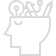Others